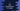# C program to find out the palindrome number in a range# C program to find out the palindrome numbers in a range :

In this C programming tutorial, we will learn how to find out all palindrome numbers in a range. Palindrome numbers are numbers that look same if reversed. For example, 141 is a palindrome number but 122 is not.

Our program will take the start number and the end number as input and it will find out all palindrome numbers between these numbers including them.

To find out a palindrome, we will reverse the number and compare it with the original number. If both numbers are equal, means the number is a palindrome.

## C program :

Let’s take a look at the program :

``````#include <stdio.h>

int main()
{
//1
int startNumber;
int endNumber;
int i;
int currentNumber;
int reversedNumber;
int rem;

//2
printf("Enter the first number : ");
scanf("%d", &startNumber);

//3
printf("Enter the second number : ");
scanf("%d", &endNumber);

//4
printf("Palindrome number between %d and %d are : \n", startNumber, endNumber);

for (i = startNumber; i <= endNumber; i++)
{
currentNumber = i;
reversedNumber = 0;

//5
while (currentNumber)
{
rem = currentNumber % 10;
currentNumber = currentNumber / 10;
reversedNumber = reversedNumber * 10 + rem;
}

if(i == reversedNumber){
printf("%d ", i);
}
}
return 0;
}
``````

### Explanation :

The commented numbers in the above program denote the step numbers below :

1. First, create a few integer variables to use in the program.
2. Ask the user to enter the first number. Read and store it in the startNumber.
3. Similarly, ask the user to enter the second number. Read and store it in the endNumber variable.
4. Print out the palindrome numbers in this range. Use one for loop to scan the numbers one by one starting from startNumber up to endNumber.
5. Inside the loop, we are using one while loop to find out the reverse of the current number. If the reversed number is equal to the current number, print it. Else, move to the next number.

### Sample Output :

``````Enter the first number : 10
Enter the second number : 100
Palindrome number between 10 and 100 are :
11 22 33 44 55 66 77 88 99

Enter the first number : 1000
Enter the second number : 2000
Palindrome number between 1000 and 2000 are :
1001 1111 1221 1331 1441 1551 1661 1771 1881 1991
``````

You might also like :